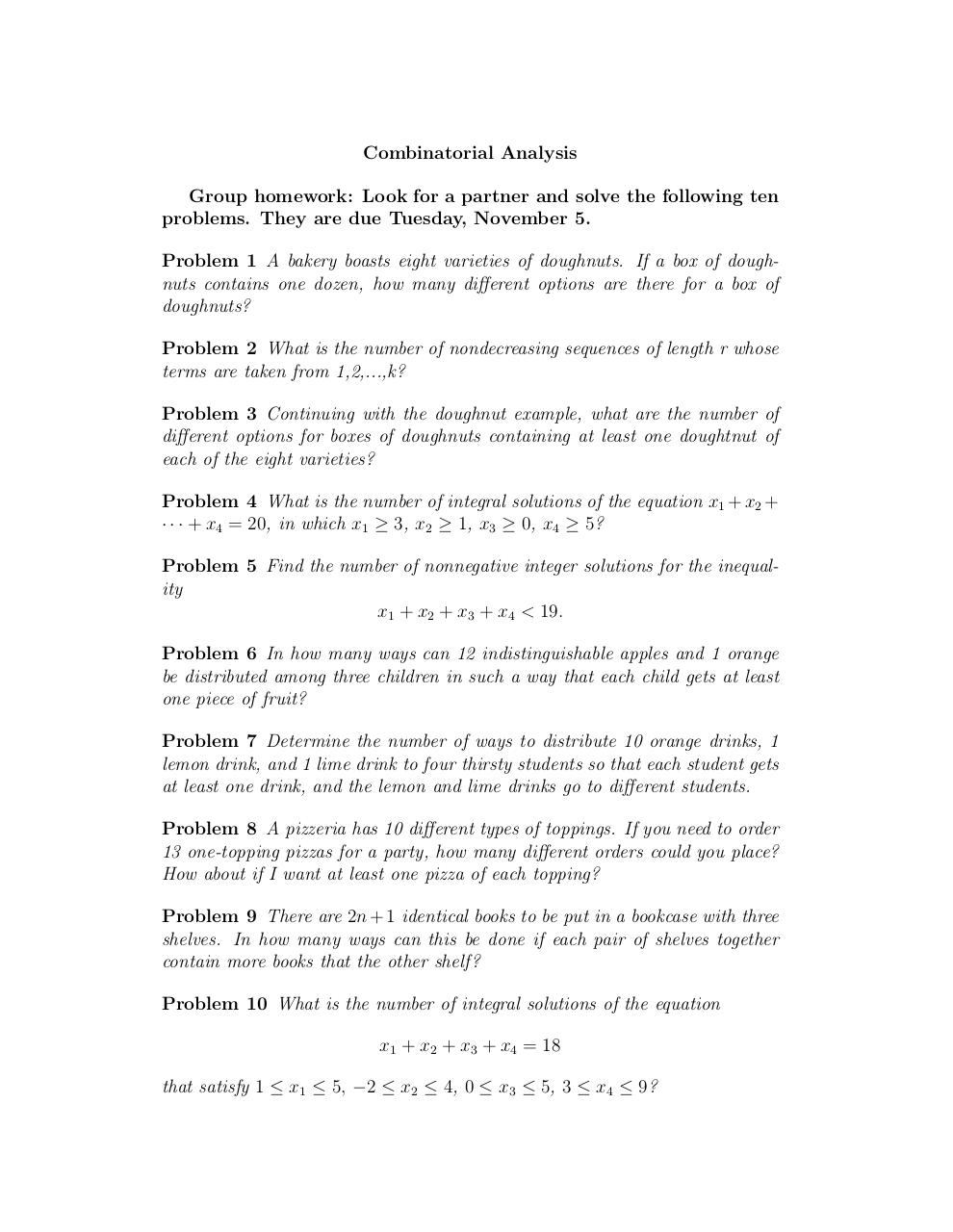# Day 9 more problems .pdf

### File information

Original filename: Day 9- more-problems.pdf

This PDF 1.4 document has been generated by TeX / pdfTeX-1.40.3, and has been sent on pdf-archive.com on 24/01/2014 at 05:06, from IP address 169.231.x.x. The current document download page has been viewed 790 times.
File size: 69 KB (1 page).
Privacy: public file

Day 9- more-problems.pdf (PDF, 69 KB)

### Document preview

Combinatorial Analysis
Group homework: Look for a partner and solve the following ten
problems. They are due Tuesday, November 5.
Problem 1 A bakery boasts eight varieties of doughnuts. If a box of doughnuts contains one dozen, how many different options are there for a box of
doughnuts?
Problem 2 What is the number of nondecreasing sequences of length r whose
terms are taken from 1,2,...,k?
Problem 3 Continuing with the doughnut example, what are the number of
different options for boxes of doughnuts containing at least one doughtnut of
each of the eight varieties?
Problem 4 What is the number of integral solutions of the equation x1 + x2 +
· · · + x4 = 20, in which x1 ≥ 3, x2 ≥ 1, x3 ≥ 0, x4 ≥ 5?
Problem 5 Find the number of nonnegative integer solutions for the inequality
x1 + x2 + x3 + x4 &lt; 19.
Problem 6 In how many ways can 12 indistinguishable apples and 1 orange
be distributed among three children in such a way that each child gets at least
one piece of fruit?
Problem 7 Determine the number of ways to distribute 10 orange drinks, 1
lemon drink, and 1 lime drink to four thirsty students so that each student gets
at least one drink, and the lemon and lime drinks go to different students.
Problem 8 A pizzeria has 10 different types of toppings. If you need to order
13 one-topping pizzas for a party, how many different orders could you place?
How about if I want at least one pizza of each topping?
Problem 9 There are 2n + 1 identical books to be put in a bookcase with three
shelves. In how many ways can this be done if each pair of shelves together
contain more books that the other shelf ?
Problem 10 What is the number of integral solutions of the equation
x1 + x2 + x3 + x4 = 18
that satisfy 1 ≤ x1 ≤ 5, −2 ≤ x2 ≤ 4, 0 ≤ x3 ≤ 5, 3 ≤ x4 ≤ 9?#### HTML Code

Copy the following HTML code to share your document on a Website or Blog

#### QR Code### Related keywords